# Even numbers

Even numbers are whole numbers that are exactly divisible by $$2.$$

Let us take $$6$$ blue-colored counters.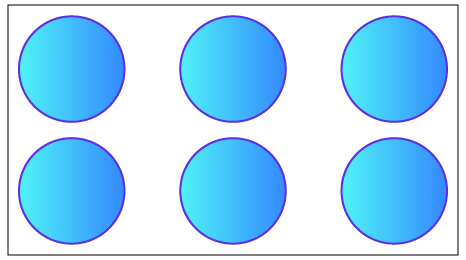Let us try and pair the counters.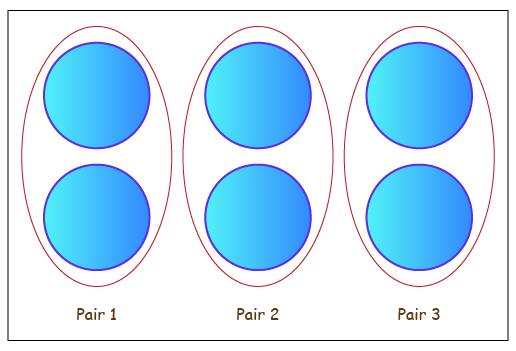We are able to pair all the $$6$$ counters and form $$3$$ pairs.
There are no counters that are left unpaired.
Thus, we can conclude that $$6$$ is an even number.

Let’s divide $$6$$ by $$2.$$
We get the quotient as $$3,$$ which is equal to the number of pairs that are formed.
We get the remainder as $$0,$$ which is equal to the number of counters that cannot be paired.

Whenever we try to pair an even number of counters, we will be left with NO counter that cannot be paired.
Whenever an even number is divided by $$2,$$ we will always get the remainder as $$0.$$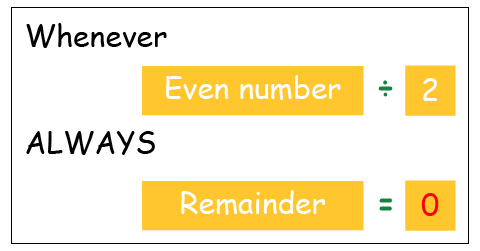Note: An even number always ends in $$0, 2, 4, 6$$ or $$8.$$

## Odd and even numbers

Let us perform addition on different combinations of odd and even numbers.

 Number $$1$$ Number $$2$$ Sum $$4$$ [Even] $$8$$ [Even] $$12$$ [Even] $$7$$ [Odd] $$3$$ [Odd] $$10$$ [Even] $$1$$ [Odd] $$6$$ [Even] $$7$$ [Odd] $$2$$ [Even] $$9$$ [Odd] $$11$$ [Odd]

Let us summarize the result.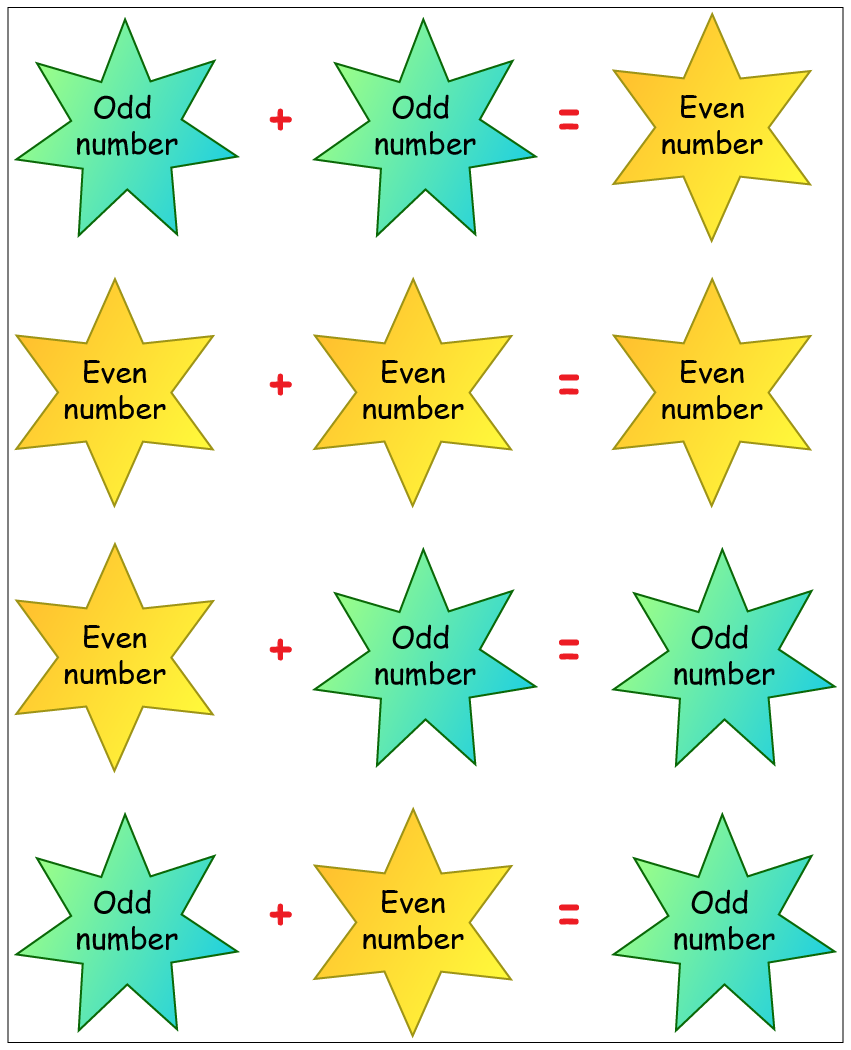You can also learn the properties of ODD and EVEN numbers, the CUEMATH way with the help of this simple video!

## Solved Examples

### Example 1:

Find whether the number of objects given in the box is odd or even.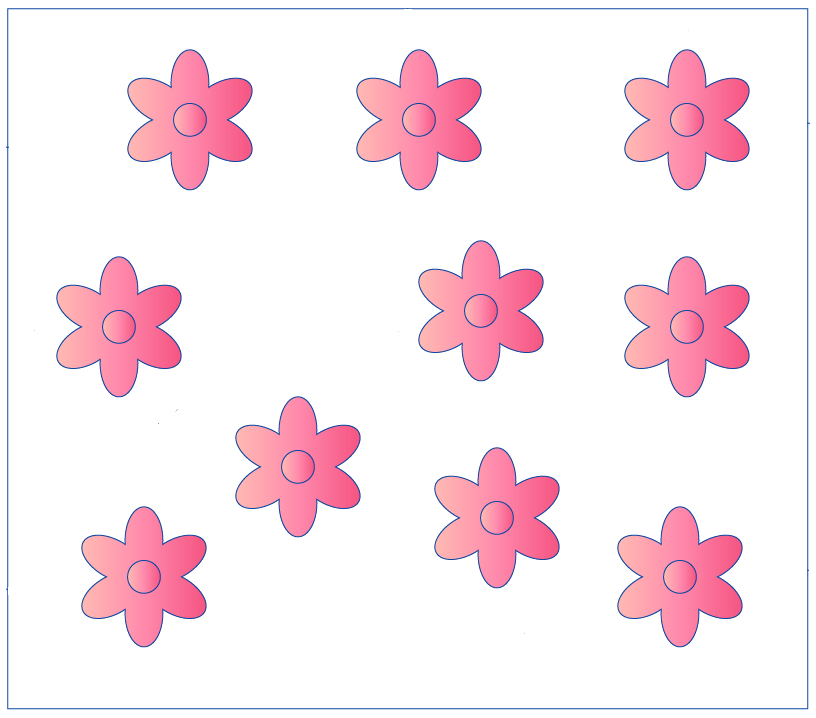Step 1:

Count the number of objects.
Number of flowers $$= 10$$

Step 2:

Pair the objects.
As shown below, there are $$5$$ pairs of flowers.
All the flowers have been paired.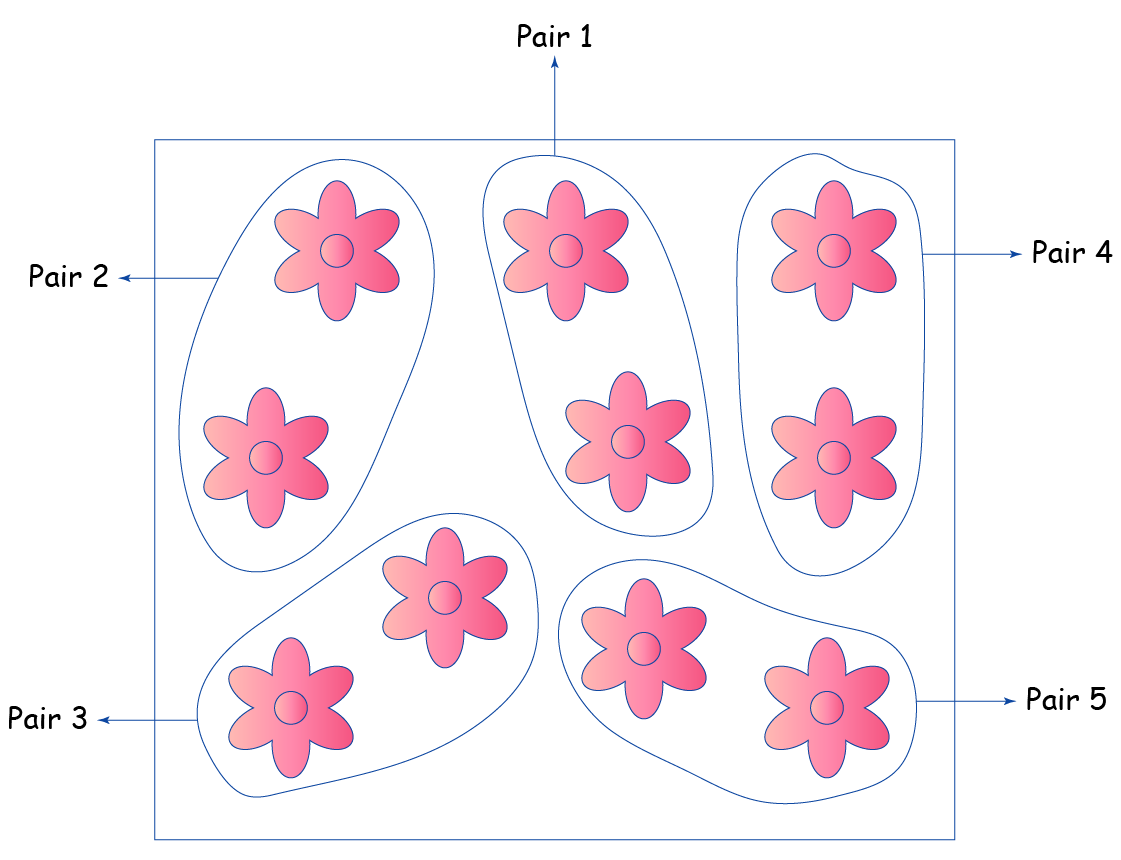Step 3:

If all the objects can be paired then the number of objects represents an EVEN number.

Thus, $$10$$ is an EVEN number.

## Practice Questions

(1)  Circle the even numbers
(a)  $$27\qquad74\qquad68\qquad84\qquad98$$
(b)  $$143\quad280\quad342\quad515\quad742$$
(c)  $$1001\quad3202\quad4009\quad6184\quad3866$$
(d)  $$7172\quad5490\quad3381\quad8769\quad9999$$

Hint: An even number always ends in $$0, 2, 4, 6$$ or $$8.$$

(2)  Which two digits of the number $$7253$$ must be interchanged to make it an even number?

Hint: An even number always ends in $$0, 2, 4, 6$$ or $$8.$$

(3)  Which of these numbers when added to an odd number results in an even number?

(a)  $$21$$         (b)  $$42$$         (c)  $$68$$         (d)  $$102$$

Hint: Odd $$+$$ Odd $$=$$ Even

More Important Topics
Numbers
Algebra
Geometry
Measurement
Money
Data
Trigonometry
Calculus
More Important Topics
Numbers
Algebra
Geometry
Measurement
Money
Data
Trigonometry
Calculus
Learn from the best math teachers and top your exams

• Live one on one classroom and doubt clearing
• Practice worksheets in and after class for conceptual clarity
• Personalized curriculum to keep up with school